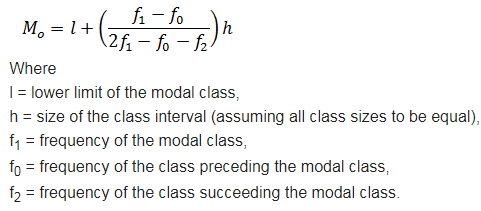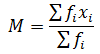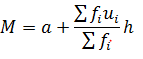# NCERT Solutions for Class 10 Maths:Chapter 14 Statistics Exercise 14.2

In this page we have NCERT Solutions for Class 10 Maths:Chapter 14 Statistics for Exercise 14.2 . Hope you like them and do not forget to like , social_share and comment at the end of the page.
Question 1
The following table shows the ages of the patients admitted in a hospital during a year

 Age (in years) 5-15 15-25 25-35 35-45 45-55 55-65 Number of patients 6 11 21 23 14 5

Find the mode and the mean of the data given above. Compare and interpret the two
measures of central tendency.
Solutionodal class = 35 – 45, l = 35, class width (h) = 10, f1 = 23, f0 = 21 and f2 = 14
Substituting the values, we get
ode= 36.8 year
Now Calculation of Mean
 Class Interval Frequency (fi) Mid-point (xi) fixi 5-15 6 10 60 15-25 11 20 220 25-35 21 30 630 35-45 23 40 920 45-55 14 50 700 55-65 5 60 300 ∑ fi = 80 ∑ fixi = 2830= 2830/80 = 35.37 yr
So, Maximum number of patients are of age 36.8 year and Mean age of the patient is 35.37 year
Question 2
The following data gives the information on the observed lifetimes (in hours) of 225
electrical components:

 Lifetime (in hours) 0-20 20-40 40-60 60-80 80-100 100-120 Frequency 10 35 52 61 38 29

Determine the modal lifetimes of the components.
Solutionodal class of the given data is 60–80.
odal class = 60-80, l = 60, f1 = 61, f0 = 52, f2 = 38 and h = 20
Substituting these values, we get
ode = 65,625

Question 3
The following data gives the distribution of total monthly household expenditure of 200
families of a village. Find the modal monthly expenditure of the families. Also, find the
mean monthly expenditure:

 Expenditure Number of families 1000-1500 24 1500-2000 40 2000-2500 33 2500-3000 28 3000-3500 30 3500-4000 22 4000-4500 16 4500-5000 7

Solutionodal class = 1500-2000, l = 1500, f1 = 40, f0 = 24, f2 = 33 and h = 500
Substituting these values, we get
ode= Rs 1847.83
Calculation for mean

 Class Interval fi xi di = xi - a ui = di/h fi ui 1000-1500 24 1250 -1500 -3 -72 1500-2000 40 1750 -1000 -2 -80 2000-2500 33 2250 -500 -1 -33 2500-3000 28 2750 0 0 0 3000-3500 30 3250 500 1 30 3500-4000 22 3750 1000 2 44 4000-4500 16 4250 1500 3 48 4500-5000 7 4750 2000 4 28 ∑ fi  = 200 ∑fiui = -35= 2750 + (35/200) ? 500
= 2750 - 87.50 = 2662.50

Question 4
The following distribution gives the state-wise teacher-student ratio in higher secondary
schools of India. Find the mode and mean of this data. Interpret the two measures.
 Number of students per teacher Number of states/U.T 15 − 20 3 20 -  25 8 25 - 30 9 30 - 35 10 35 - 40 3 40 – 45 0 45 – 50 0 50 - 55 2

Solutionodal class = 30-35, l = 30, f1 = 10, f0 = 9, f2 = 3 and h = 500
Substituting these values, we get
ode= 30.6
Calculation for mean  by step deviation method ( Assumed mean 32.5)

 Class Interval fi xi di = xi - a ui = di/h fi ui 15 − 20 3 17.5 -15 -3 -9 20 -  25 8 22.5 -10 -2 -16 25 - 30 9 27.5 -5 -1 -9 30 - 35 10 32.5 0 0 0 35 - 40 3 37.7 5 1 3 40 – 45 0 42.5 10 2 0 45 – 50 0 47.5 15 3 0 50 - 55 2 52.5 20 4 8 ∑ fi  = 35 ∑fiui = -23= 29.2

Question 5
The given distribution shows the number of runs scored by some top batsmen of the
world in one-day international cricket matches.
 Runs scored Number of batsmen 3000-4000 4 4000-5000 18 5000-6000 9 6000-7000 7 7000-8000 6 8000-9000 3 9000-1000 1 1000-1100 1
Find the mode of the data.
SolutionFrom the given data, it can be observed that the maximum class frequency is 18,
belonging to class interval 4000 − 5000. Therefore, modal class = 4000 − 5000
Lower limit (l) of modal class = 4000
Frequency (f1) of modal class = 18
Frequency (f0) of class preceding modal class = 4
Frequency (f2) of class succeeding modal class = 9
Class size (h) = 1000
Substituting these values, we get
ode =4608.7 runs

Question 6
A student noted the number of cars passing through a spot on a road for 100 periods each of 3 minutes and summarized it in the table given below. Find the mode of the data

 Number of cars 0-10 10-20 20-30 30-40 40-50 50-60 60-70 70-80 Frequency 7 14 13 12 20 11 15 8

SolutionHere maximum class frequency is 20, belonging to 40 − 50 class intervals. Therefore, modal class = 40 − 50
Lower limit (l) of modal class = 40
Frequency (f1) of modal class = 20
Frequency (f0) of class preceding modal class = 12
Frequency (f2) of class succeeding modal class = 11
Substituting these values, we get
ode =44.7

link to this page by copying the following text
Reference Books for class 10

Given below are the links of some of the reference books for class 10 math.

You can use above books for extra knowledge and practicing different questions.

### Practice Question

Question 1 What is $1 - \sqrt {3}$ ?
A) Non terminating repeating
B) Non terminating non repeating
C) Terminating
D) None of the above
Question 2 The volume of the largest right circular cone that can be cut out from a cube of edge 4.2 cm is?
A) 19.4 cm3
B) 12 cm3
C) 78.6 cm3
D) 58.2 cm3
Question 3 The sum of the first three terms of an AP is 33. If the product of the first and the third term exceeds the second term by 29, the AP is ?
A) 2 ,21,11
B) 1,10,19
C) -1 ,8,17
D) 2 ,11,20

Note to our visitors :-

Thanks for visiting our website.
DISCLOSURE: THIS PAGE MAY CONTAIN AFFILIATE LINKS, MEANING I GET A COMMISSION IF YOU DECIDE TO MAKE A PURCHASE THROUGH MY LINKS, AT NO COST TO YOU. PLEASE READ MY DISCLOSURE FOR MORE INFO.